Processing ......FreeComputerBooks.com Links to Free Computer, Mathematics, Technical Books all over the World

Algorithms for Optimization
Top Free Data Science Books 🌠 - 100% Free or Open Source!
• Title Algorithms for Optimization
• Author(s) Mykel J. Kochenderfer, Tim A. Wheeler
• Publisher: The MIT Press; Illustrated edition (March 12, 2019); eBook (Creative Commons Licensed)
• Hardcover: 520 pages
• eBook: PDF and ePub
• Language: English
• ISBN-10: 0262039427
• ISBN-13: 978-0262039420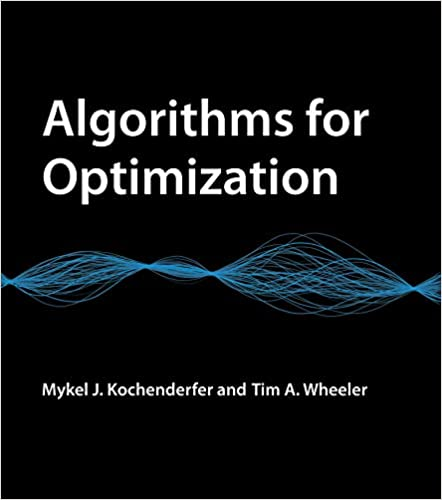Book Description

A comprehensive introduction to optimization with a focus on practical algorithms for the design of engineering systems.

This book offers a comprehensive introduction to optimization with a focus on practical algorithms. The book approaches optimization from an engineering perspective, where the objective is to design a system that optimizes a set of metrics subject to constraints.

• Mykel J. Kochenderfer is Assistant Professor in the Department of Aeronautics and Astronautics at Stanford University and the author of Decision Making Under Uncertainty: Theory and Application.
• Tim A. Wheeler wrote his PhD thesis on safety validation for autonomous vehicles and is now in industry working on air taxis.
Reviews, Ratings, and Recommendations: Related Book Categories: Read and Download Links:Similar Books:
•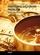Traveling Salesman Problem, Theory and Applications

This book is a collection of current research in the application of evolutionary algorithms and other optimal algorithms to solving the Travelling Salesman Problem (TSP). Most importantly, it presents both theoretical as well as practical applications of TSP,

•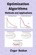Optimization Algorithms- Methods and Applications

This book covers state-of-the-art optimization methods and their applications in wide range especially for researchers and practitioners who wish to improve their knowledge in this field.

•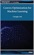Convex Optimization for Machine Learning (Changho Suh)

This book covers an introduction to convex optimization, one of the powerful and tractable optimization problems that can be efficiently solved on a computer. The goal is to help develop a sense of what convex optimization is, and how it can be used.

•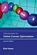Introduction to Online Convex Optimization (Elad Hazan)

This book presents a robust machine learning approach that contains elements of mathematical optimization, game theory, and learning theory: an optimization method that learns from experience as more aspects of the problem are observed.

•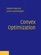Convex Optimization (Stephen Boyd, et al.)

On recognizing convex optimization problems and then finding the most appropriate technique for solving them. It contains many worked examples in fields such as engineering, computer science, mathematics, statistics, finance, and economics.

•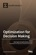Optimization for Decision Making: Linear and Quadratic Models

This book illustrates how to formulate real world problems using linear and quadratic models; It focus on developing modeling skills to support valid decision making for complex real world problems.

•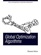Global Optimization Algorithms - Theory and Application

This book is devoted to global optimization algorithms, which are methods to find optimal solutions for given problems. It especially focuses on Evolutionary Computation by discussing evolutionary algorithms, genetic algorithms, Genetic Programming, etc.

•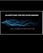Algorithms for Decision Making (Mykel Kochenderfer, et al)

This textbook provides a broad introduction to algorithms for decision making under uncertainty, covering the underlying mathematical problem formulations and the algorithms for solving them.

•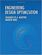Engineering Design Optimization (Joaquim R. Martins, et al)

The philosophy of this book is to provide a detailed enough explanation and analysis of optimization methods so that readers can implement a basic working version. Practical tips are included for common issues encountered in practical engineering design optimization.

•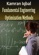Fundamental Engineering Optimization Methods (Kamran Iqbal)

This bookcovers the fundamentals of commonly used optimization methods in engineering design. These include graphical optimization, linear and nonlinear programming, numerical optimization, and discrete optimization.

•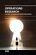Operations Research - the Art of Making Good Decisions

This book is dedicated to operations research of broad applications, it provides a tool for efficient use of natural resources. Both theory and practice of operations research and its related concepts are covered in the book.

•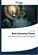Basic Queueing Theory: System Performance Modeling

Queueing Theory is one of the most commonly used mathematical tool for the performance evaluation of systems. The aim of the book is to present the basic methods, approaches in a Markovian level for the analysis of not too complicated systems.

•Knapsack Problems: Algorithms and Computer Implementations

The text fully develops an algorithmic approach to Knapsack Problems without losing mathematical rigor. It provides a comprehensive overview of the methods for solving Knapsack Problems (KP), its variants and generalizations.

Book Categories
 :All CategoriesTop Free BooksRecent BooksMiscellaneous BooksComputer EngineeringComputer LanguagesComputer ScienceData Science/DatabasesJava and Java EE (J2EE)Linux and UnixMathematicsMicrosoft and .NETMobile ComputingNetworking and CommunicationsSoftware EngineeringSpecial TopicsWeb Programming
Other Categories